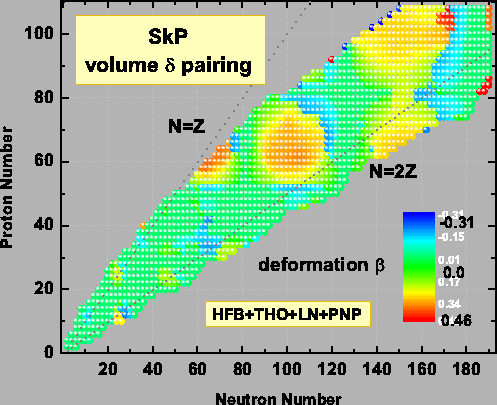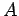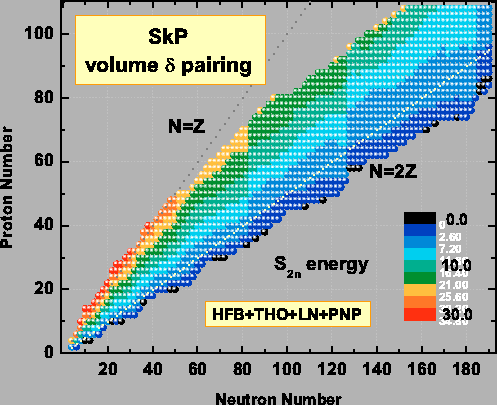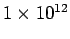Next: Transformed Harmonic Oscillator Basis Up: Large-Scale Self-Consistent Nuclear Mass Previous: Variational Equations

# Large-Scale Microscopic Nuclear Mass Calculations

The large-scale microscopic nuclear mass calculations, such as those of Refs. [13,20,21,22], typically require that the variational equations are repeatedly solved for thousands of nuclei. For example, when adjusting the parameters of the energy density functional to measured masses, one has to calculate ground-state configurations of around two-thousand nuclei many times during the fitting process.Actually, the situation is even more complicated, as several independent calculations have to be carried out for a given nucleus to find the ground-state energy of the system among several coexisting local minima. Furthermore, if odd-and odd-odd nuclei are considered during the fitting process, many one-quasiparticle and two-quasiparticle states have to be considered to find the actual ground state. Finally, when the functional has been established, properties of around ten-thousand particle-bound nuclei throughout the nuclear chart can be computed. All in all, fitting a functional and preparing a mass table is a challenging computational problem that requires highly optimized numerical codes and excellent utilization of modern multiprocessor computer resources.Our group has been laying out theoretical foundations and constructing computational tools to tackle this ambitious task. We utilize a fast HFBRAD code for spherical HFB calculations , which takes no more than 10 CPU minutes per nucleus on an Intel Xeon 2.8 GHz processor, as well as the HFBTHO code for axially deformed HFB calculations [24,25] with acceptable processor speed - averaging to about 1 CPU hour per nucleus.

The large-scale mass calculations based on the HFB+THO code, extended with a minimal MPI communication in order to run in a parallel regime across the nodes of the multiprocessor computer, are illustrated in Figs. 1 and 2, which display, respectively, calculated charts of nuclear deformations and two-neutron separation energies for particle-bound even-even nuclei. We used the SkP energy functional , which has a general form given by Eqs. (4) and (5). Our load-balancing routine, which scales the problem to 200 processors, allows us to perform these calculations in a single 24 wall-clock hour run on a 4 Tflop machine Cheetah at ORNL (1 Tflop=floation-point operations/sec) . For the details of the Skyrme-HFB deformed nuclear mass table with SLy4 and SkP energy density functionals, see Ref. . In the following, some particular aspects of our Skyrme-DFT calculations are briefly discussed.

SubsectionsNext: Transformed Harmonic Oscillator Basis Up: Large-Scale Self-Consistent Nuclear Mass Previous: Variational Equations
Jacek Dobaczewski 2006-01-17